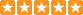# Search

Show:
Sort By:
INTRODUCTION DFRobot Gravity: Analog pH meter V2 is specifically designed to measure the pH of ..
₹3,759.00
INTRODUCTION The Analog Signal Isolator can isolate analog signal and provide isolated power to..
₹1,899.00
INTRODUCTION This is an Arduino compatible sound level meter(also known as decibel meter, noise..
₹3,759.00
INTRODUCTION This spear tip pH sensor/meter kit can directly stab into semisolid material to me..
₹9,409.00
INTRODUCTION This is an Arduino-compatible TDS sensor/Meter Kit for measuring TDS value of the ..
₹1,229.00
INTRODUCTION The turbidity sensor detects water quality by measuring level of turbidity. It is ab..
₹949.00INTRODUCTION The ML8511 is a UV sensor, which is suitable for acquiring UV intensity indoors or o..
₹949.00
INTRODUCTION This is a water pressure sensor adopts DFRobot Gravity 3-pin interface. It support..
₹1,559.00
INTRODUCTION What's the difference between Pi and Arduino? We all know Raspberry Pi is a m..
₹1,899.00
Introduction Gravity: CCS811 Air Quality Sensor can measure the eCO2 (equival..
₹1,479.00
INTRODUCTION The Gravity Conductivity Sensor Switch module can be used to detect if an object h..
₹339.00
INTRODUCTION DFRobot's latest generation of digital DHT11 Temperature and Humidity sensor is ..
₹499.00
INTRODUCTION On the same I2C port, DFRobot Gravity: I2C Multiplexer solves the address conflict..
₹659.00
INTRODUCTION Introducing the Gravity Digital Relay Module – the latest addition to DFRobot&..
₹379.00
INTRODUCTION This is an Arduino digital infrared distance sensor. It has an adjustable detectio..
₹1,229.00